﻿ Reflecting Property of an Ellipse

# Reflecting Property of an Ellipse

LESSON READ-THROUGH (Part 1 of 2)
by Dr. Carol JVF Burns (website creator)
Follow along with the highlighted text while you listen!
• PRACTICE (online exercises and printable worksheets)

Ellipses were introduced in Introduction to Conic Sections, as one of several different curves (‘conic sections’) that are formed by intersecting a plane with an infinite double cone.

The prior section explores the standard definition of an ellipse. The essential ideas are reviewed below for your convenience.

The purpose of this section is to explore the reflecting property of ellipses—that light emitted from one focus is reflected through the other focus.

## Review: Definition of an Ellipse

• An ellipse is determined by three pieces of information: two points and a number.
• The two points are often named $\,F_1\,$ and $\,F_2\,,$ and are called the foci (pronounced FOE-sigh) of the ellipse.
(The singular form of foci is focus: one focus, two foci.)
• The number is often denoted by $\,k\,$;
it must be strictly greater than the distance between the foci.
That is, $\,k > d(F_1,F_2)\,.$
• The ellipse determined by $\,F_1\,,$ $\,F_2\,$ and $\,k\,$ is, by definition, the set of points $\,P\,$ in a plane
such that the sum of the distances to the two fixed points is constant:
$$\cssId{s14}{d(P,F_1) + d(P,F_2) = k}$$

## Reflecting Property of an Ellipse

 In an ellipse: Light emitted from one focus is reflected through the other focus. You can play with this reflecting property at right: Points $F_1\,$ and $\,F_2\,$ are the foci. They can be dragged to change the shape of the ellipse. ‘Light’ is emitted from $\,F_1\,$ (the red segment). The light hits the ellipse at point $\,\color{purple}{P}\,.$ (Point $\,P\,$ can be dragged around the ellipse.) The light is always reflected so that it passes through $\,F_2\,.$ Point $\,S\,$ can also be moved to change the shape of the ellipse. (For fixed foci, moving $\,S\,$ changes the ellipse constant.) Notes: Drag $\,F_1\,$ to coincide with $\,F_2\,,$ creating a circle. Light emitted from the center hits the circle and is reflected back through the center. If (say) a light bulb is placed at $\,F_1\,,$ then light waves go out in all directions at the same time. Click the button to get more (randomly-generated) light! (Refresh the page if you want to start over.) (refresh the page to start over)

## Two Applications of the Ellipse Reflecting Property

• Medicine:
Solid material (often referred to as ‘stones’) can sometimes form in the human body, causing serious pain.
In the gallbladder, they're called gallstones.
In the kidney or urinary tract, they're called kidney stones.
The process called lithotripsy (LITH-oh-trip-see) uses the reflecting property of the ellipse
to shatter the stones into tiny pieces, which the body can then get rid of on its own.

Shockwaves are emitted from one focus of an ellipse, with the targeted stone located at the other focus.
• Architecture:
In a whispering gallery or whispering room, quiet sounds (whispers)
emitted from one particular place in the room can be clearly heard at another (remote) place.

Different methods can achieve this effectone method uses a ceiling with elliptical cross-sections (called an ‘ellipsoid’).
Note that in a given ellipse, all the reflecting paths from one focus to the other have the same length (the ellipse constant),
so sound waves emitted at the same time will also arrive at the same time.

## Why Does the Ellipse Reflecting Property Work?

To see why light from one focus is always reflected through the other focus, we need a few ideas.

We start by looking at a simple childhood game:

### ‘Touch the Wall’ Playground Game

 This game involves a wall (or fence) and two special spots marked on the ground. Two or more kids must run from one designated spot ($\,P_1\,$), touch the wall ($W$), and then run to a second designated spot ($P_2$). First arriver at $\,P_2\,$ wins the game! Of course, your running speed matters. But, the distance that you run also matters. To maximize your chances of winning, where should you touch the wall? Does it matter? You can ‘play with’ this game above: Drag point $\,W\,$ along the wall, keeping it between the two yellow points. The red path is the distance you must run; its length is displayed at the top. You'll see that different paths have different lengths. Let $\,m_1\,$ and $\,m_2\,$ denote the measures of the indicated angles: $\,m_1\,$ is the angle between $\,\overline{P_1W}\,$ (the line segment from $\,P_1\,$ to $\,W\,$) and the wall that lies to the left of $\,W\,$ $\,m_2\,$ is the angle between $\,\overline{P_2W}\,$ (the line segment from $\,P_2\,$ to $\,W\,$) and the wall that lies to the right of $\,W\,$ These angle measures, in degrees, are also displayed. By dragging $\,W$, try to make the length of the red path as small as possible. What do you notice about the relationship between $\,m_1\,$ and $\,m_2\,$? For another direction: Try to get $\,m_1\,$ as close to $\,m_2\,$ as possible. What do you notice about the length of the red path? You can move points $\,P_1\,$ and $\,P_2\,$ to change where the kids start and stop. However, keep $\,P_1\,$ to the left of $\,P_2\,.$ You can drag the two yellow points to change the location of the wall.

### Minimizing the Length of the Path from a Point, to a Line, to a Second Point on the Same Side of the Line

Let's summarize and prove the results of the prior exploration.

Suppose you have a line, and two different points on the same side of the line.

The shortest path
• from one point ($\,P_1\,$)
• to the line ($\,W\,$)
• to the second point ($\,P_2\,$)
is when the two line segments make equal angles with the line ($\,m_1 = m_2\,$).

Indeed, the following two statements are equivalent:
• $\,d(P_1,W) + d(P_2,W)\,$ is minimized over all points $\,W\,$ on the line
• $\,m_1 = m_2\,$
If one statement is true, so is the other.
If one statement is false, so is the other.

For ease of reference in what follows,
this equivalence is referred to as the ‘Point/Line/Point Equivalence’.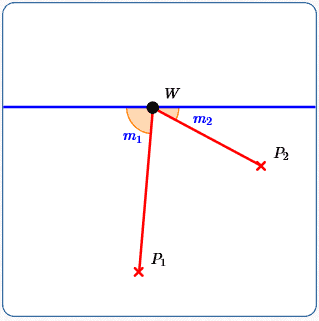### Proof of the Point/Line/Point Equivalence

The Point/Line/Point Equivalence is easy to prove, with a bit of cleverness.

As shown at right, reflect $\,\overline{P_2W}\,$ about the line;
call the end of the new (reflected) segment $\,P_2'\,.$

The path from $\,P_1\,$ to $\,W\,$ to $\,P_2\,$
has the same length as
the path from $\,P_1\,$ to $\,W\,$ to $\,P_2'\,.$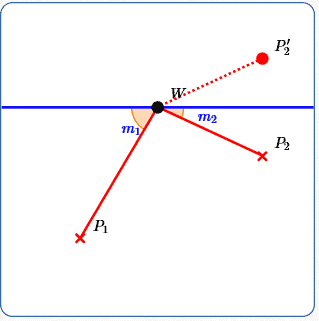Now it's clear how to minimize the distance $\,d(P_1,W) + d(P_2,W)\,$:
• Since the shortest distance between two points is a straight line,
make a straight-line segment from $\,P_1\,$ to $\,W\,$ to $\,P_2'\,.$
• Since vertical angles have equal measures, $\,m_1 = m_3\,.$
• As a reflected angle, $\,m_3 = m_2\,.$
• Thus, $\,m_1 = m_2\,.$
For the other direction:
• Suppose $\,m_1 = m_2\,.$
As reflected angles, $\,m_2 = m_3\,.$
Thus, $\,m_1 = m_3\,.$
• With $\,m_1 = m_3\,,$ the segment from $\,P_1\,$ to $\,W\,$ to $\,P_2'\,$ is a straight line segment.
Thus, $\,d(P_1,W) + d(P_2',W)\,,$ which is equal to $\,d(P_1,W) + d(P_2,W)\,,$ is as small as possible.
Done!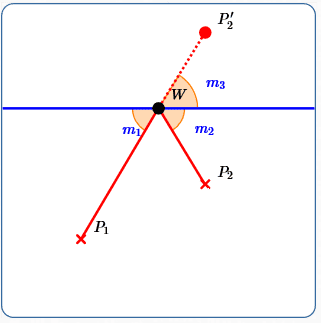### Physics Principle: the Law of Reflection

 Mother Nature is extremely efficient! When light (or sound) travels between two points, it always takes the fastest route. (For light, this is called Fermat's Principle.) In particular, this is true of reflected light. If the light/sound is always traveling through the same medium (like air), then the fastest route is also the shortest route. The well-known physics Law of Reflection states that when light/sound is reflected, the angles made with the reflecting surface must be equal. By the Point/Line/Point Equivalence, equal angles minimizes the distance $\,d(P_1,W) + d(P_2,W)\,$ over all points $\,W\,$ on the line. Make sure you understand what is being said here! You can certainly draw line segments that go from $\,P_1\,$ to $\,W\,$ to $\,P_2\,,$ where $\,m_1 \ne m_2\,.$ However, this wouldn't be a picture of reflected light. If it's reflected light, then the angles must be the same!$\,m_1\ne m_2\,$ definitely NOT reflected light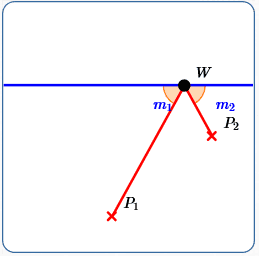reflected light $\,m_1 = m_2\,$ (minimizing the length of the red path over all points $\,W\,$ on the line)

### Sum of Distances to Foci for Points Inside, Outside, and On an Ellipse

Here's the final idea that's needed, before putting everything together.

 Consider an ellipse with ellipse constant $\,k\,$ and foci $\,F_1\,$ and $\,F_2\,.$ Let $\,P\,$ be a typical point in the plane containing the ellipse. When $\,P\,$ is ON the ellipse, $\,d(F_1,P) + d(F_2,P) = k\,.$ This is true by the definition of an ellipse. When $\,P\,$ is OUTSIDE the ellipse, $\,d(F_1,P) + d(F_2,P) > k\,.$ It's also true that: When $\,P\,$ is INSIDE the ellipse, $\,d(F_1,P) + d(F_2,P) < k\,.$ However, this fact isn't needed to prove the ellipse reflecting property.

These facts are fairly intuitive:
small changes in point locations should produce small changes in the distances involved.

Also, you can see these facts ‘in action’ in the exploration above:
drag the point $\,P\,$ outside/on/inside the ellipse, observing the displayed sum of the distances.
If desired, move the foci and/or change the ellipse constant via the slider, and explore again!

The facts are also easy to prove, as follows.

 Let $\,P\,$ be outside an ellipse with ellipse constant $\,k\,,$ as shown at right. Then: traveling from $\,F_1\,$ to $\,P\,$ to $\,F_2\,$ is longer than traveling from $\,F_1\,$ to $\,I\,$ to $\,F_2\,$ (which equals $\,k\,$). Here are the details: Let $\,I\,$ be the point where $\,\overline{PF_1}\,$ intersects the ellipse. Since $\,I\,$ is on the ellipse, $\,d(F_1,I) + d(F_2,I) = k\,.$ Since the shortest distance between two points is a straight line: $$\cssId{sb46}{d(I,P) + d(F_2,P) > d(F_2,I)}$$ Combining results: \begin{alignat}{2} \cssId{sb48}{d(F_1,P) + d(F_2,P)}\ &\cssId{sb49}{= \bigl(\,d(F_1,I) + d(I,P)\,\bigr) + d(F_2,P)} &\qquad & \cssId{sb50}{\text{break \,\overline{F_1P}\, into two pieces}}\cr\cr &\cssId{sb51}{= d(F_1,I) + \bigl(\,d(I,P) + d(F_2,P)\,\bigr)}&&\cssId{sb52}{\text{regroup}}\cr\cr &\cssId{sb53}{> d(F_1,I) + d(F_2,I)} &&\cssId{sb54}{\text{use (3)}}\cr\cr &\cssId{sb55}{= k} &&\cssId{sb56}{\text{use (2)}} \end{alignat} NOTE: The formatting \begin{align} A &= B\cr &= C\cr &> D\cr &= E \end{align} is a shorthand for $$A = B\ \ \text{and}\ \ B = C\ \ \text{and}\ \ C > D\ \ \text{and}\ \ D = E$$ from which one concludes that $\,A > E\,.$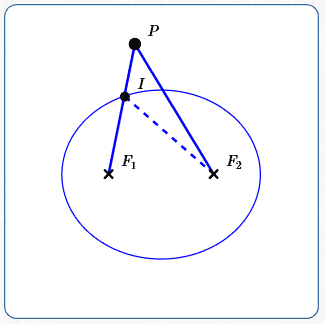Next, let $\,P\,$ be inside an ellipse with ellipse constant $\,k\,,$ as shown at right. Then: traveling from $\,F_1\,$ to $\,P\,$ to $\,F_2\,$ is shorter than traveling from $\,F_1\,$ to $\,I\,$ to $\,F_2\,$ (which equals $\,k\,$). Here are the details: Let $\,I\,$ be the point where $\,\overrightarrow{F_1P}\,$ intersects the ellipse. Since $\,I\,$ is on the ellipse, $\,d(F_1,I) + d(F_2,I) = k\,.$ Since the shortest distance between two points is a straight line: $$\cssId{sb65}{d(F_2,P) < d(P,I) + d(F_2,I)}$$ Combining results: \begin{alignat}{2} \cssId{sb67}{d(F_1,P) + d(F_2,P)}\ &\cssId{sb68}{< d(F_1,P) + \bigl(\,d(P,I) + d(F_2,I)\,\bigr)}&\qquad &\cssId{sb69}{\text{use (3)}}\cr\cr &\cssId{sb70}{= \bigl(\,d(F_1,P) + d(P,I)\,\bigr) + d(F_2,I)}&\qquad&\cssId{sb71}{\text{regroup}}\cr\cr &\cssId{sb72}{= d(F_1,I) + d(F_2,I)} &&\cssId{sb73}{\text{combine two pieces to get \,\overline{F_1I}\,}}\cr\cr &\cssId{sb74}{= k}&&\cssId{sb75}{\text{use (2)}} \end{alignat} NOTE: The formatting \begin{align} A &< B\cr &= C\cr &= D\cr &= E \end{align} is a shorthand for $$A < B\ \ \text{and}\ \ B = C\ \ \text{and}\ \ C = D\ \ \text{and}\ \ D = E$$ from which one concludes that $\,A < E\,.$### Putting It All Together

With all the prior facts in place, it's now easy to understand the ellipse reflecting property.
It must be shown that light emitted from one focus is reflected through the other focus.

Refer to the sketch at right as you read.

 Consider an ellipse with foci $\,F_1\,$ and $\,F_2\,$ and ellipse constant $\,k\,.$ Suppose that light is emitted from $\,F_1\,$ and hits the ellipse at an arbitrary point $\,P\,.$ Draw the tangent line to the ellipse at $\,P\,.$ All points on this tangent line, except $\,P\,$ itself, lie outside the ellipse. This tangent line is the ‘wall’ off of which light reflects. As shown at right, let $\,m_1\,$ denote the measure of the angle between $\,\overline{F_1P}\,$ and the tangent line. By the Law of Reflection, reflected light must create equal angles with this tangent line. As shown at right, let $\,m_2\,$ denote the measure of the angle between $\,\overline{F_2P}\,$ and the tangent line. It suffices to show that $\,m_1 = m_2\,.$ In other words, it suffices to show that the angle that $\,\overline{F_2P}\,$ creates with the tangent line is consistent with the behavior of reflected light. By the ellipse property, $\,d(F_1,P) + d(F_2,P) = k\,.$ Let $\,Q\,$ denote a point on the tangent line. For $\,Q\ne P\,,$ $\,Q\,$ is outside the ellipse, so that $\,d(F_1,Q) + d(F_2,Q) > k\,.$ Therefore, for every point $\,Q\,$ on the tangent line: $$\cssId{sb97}{\overbrace{d(F_1,P) + d(F_2,P)}^{=\ k} \le \overbrace{d(F_1,Q) + d(F_2,Q)}^{\ge\ k}}$$ Therefore, point $\,P\,$ minimizes the sum $\,d(F_1,Q) + d(F_2,Q)\,$ over the entire tangent line. By the Point/Wall/Point Equivalence, $\,m_1 = m_2\,.$ Thus, the path from $\,F_1\,$ to $\,P\,$ to $\,F_2\,$ is the path that reflected light takes, which is what we set out to show!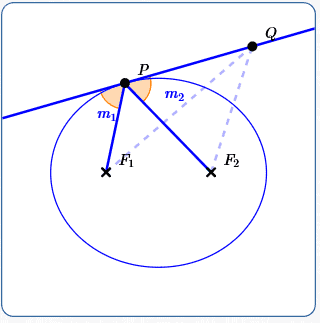Master the ideas from this section
Equations of Ellipses in Standard Form: Foci on the $x$-axis